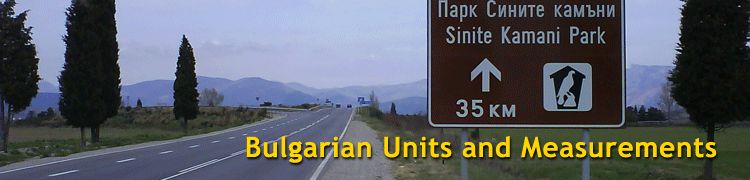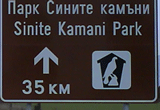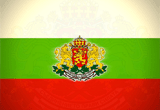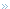Bulgarian Units and MeasurementsGeneral Information

Bulgaria uses the Metric system. In case you are not familiar with the metric system, we have prepared an Unit Converter that you can use to get an idea about measurements in Bulgaria.

 Bulgaria uses the Metric system. If you are not familiar with the metric system, we have prepared an Unit Converter that you can use to convert measurements LENGTH MEASUREMENTS CONVERT FROM   < Select >             Kilometers Miles Yards Millimeters Centimeters Inches Feet Meters CONVERT TO   < Select >             Kilometers Miles Yards Millimeters Centimeters Inches Feet Meters AREA MEASUREMENTS CONVERT FROM  < Select >  Square Kilometers Hectares Square Meters Square Miles Square Yards Square Feet Acres CONVERT TO   < Select >  Square Kilometers Hectares Square Meters Square Miles Square Yards Square Feet Acres WEIGHT MEASUREMENTS CONVERT FROM  < Select >              Kilograms Grams Pounds Ounces CONVERT TO   < Select >              Kilograms Grams Pounds Ounces

Tables of Metric Weights and Measures

 Linear Measurements 10 millimeters (mm) = 1 centimeter (cm) 10 centimeters = 1 decimeter (dm) = 100 millimeters 10 decimeters = 1 meter (m) = 1,000 millimeters 10 meters = 1 dekameter (dam) 10 dekameters = 1 hectometer (hm) = 100 meters 10 hectometers = 1 kilometer (km) = 1,000 metersArea Measurements 100 square millimeters (mm2) = 1 sq centimeter (cm2) 10,000 square centimeters = 1 sq meter (m2) = 1,000,000 sq millimeters 100 square meters = 1 are (a) 100 ares = 1 hectare (ha) = 10,000 sq meters 100 hectares = 1 sq kilometer (km2) = 1,000,000 sq metersVolume Measurements 10 milliliters (ml) = 1 centiliter (cl) 10 centiliters = 1 deciliter (dl) = 100 milliliters 10 deciliters = 1 liter (l) = 1,000 milliliters 10 liters = 1 dekaliter (dal) 10 dekaliters = 1 hectoliter (hl) = 100 liters 10 hectoliters = 1 kiloliter (kl) = 1,000 litersCubic Measurements 1,000 cubic millimeters (mm3) = 1 cu centimeter (cm3) 1,000 cubic centimeters = 1 cu decimeter (dm3) = 1,000,000 cu millimeters 1,000 cubic decimeters = 1 cu meter (m3) = 1 stere = 1,000,000 cu centimeters = 1,000,000,000 cu millimetersWeight Measurements 10 milligrams (mg) = 1 centigram (cg) 10 centigrams = 1 decigram (dg) = 100 milligrams 10 decigrams = 1 gram (g) = 1,000 milligrams 10 grams = 1 dekagram (dag) 10 dekagrams = 1 hectogram (hg) = 100 grams 10 hectograms = 1 kilogram (kg) = 1,000 grams 1,000 kilograms = 1 metric ton (t)Featured DistrictSliven
 Invest Bulgaria ChartsDomestic Credit of BulgariaNet Domestic Assets of BulgariaSee all charts
 Did You Know?Bulgarian Head NodWhen Bulgarians nod their heads up and down, they mean NO. When they move their heads from side to ... More© Copyright 2004-2021 Invest Bulgaria Group Ltd, Sofia, Bulgaria. All rights reserved.

Please read Conditions of Use, Copyright Statement and Privacy Policy for this web page and web site.Лимузини под наемElectric and Hybrid Modules RepairSEO оптимизацияBulgaria TranslationBulgarian AgricultureBulgarian TextileBestFlowers.bgSofia Web WorksBulgarian CoinsIntelligence Pathways Bulgarian Outsourcing

Crafted by Sofia Web Works. Maintained by Website Maintenance, the kings of Website Migration.

 Our Featured Members